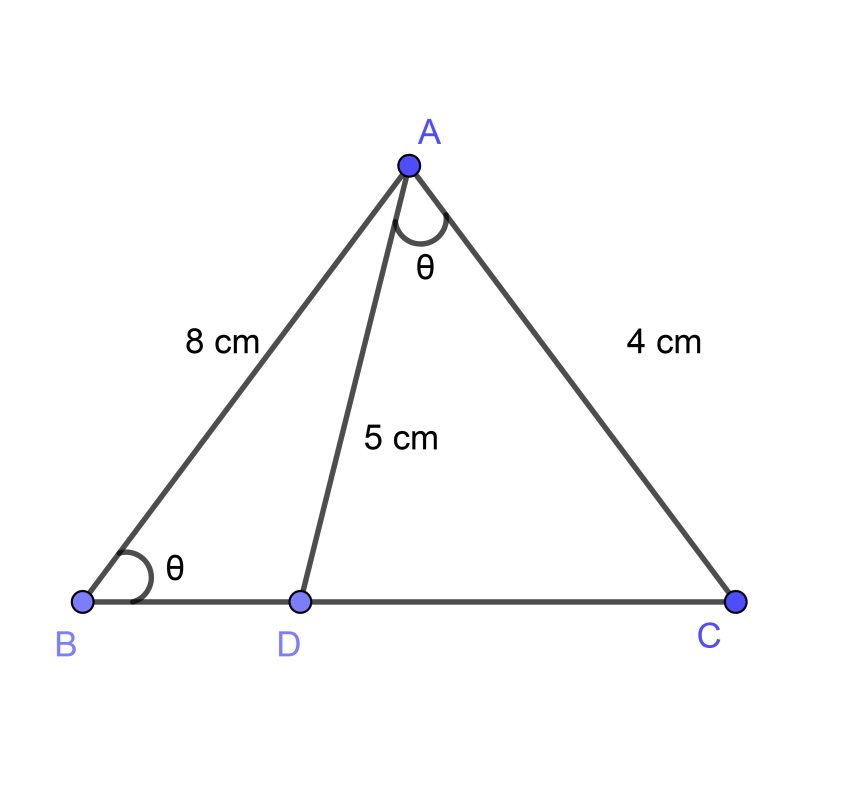Courses
Courses for Kids
Free study material
Free LIVE classes
MoreLIVE
Join Vedantu’s FREE Mastercalss

# In $\Delta ABC$, $\angle ABC=\angle DAC$, AB = 8 cm, AC = 4 cm, AD = 5 cm. Prove that $\Delta ACD$ is similar to $\Delta BCA$.Verified
361.2k+ views
Hint: Use the concept of ‘A-A similarity’ i.e. “If two angles of one triangle are congruent to two angles of another triangle, then the triangles are similar” to prove the similarity.

To solve the above problem we will draw a diagram with given measures and notations as follows,As we are done with diagram therefore we will write the given data now,
$\angle ABC=\angle DAC$,
Therefore assume $\angle ABC=\angle DAC=\theta$ for simplification
AB = 8 cm,
AC = 4 cm,
Now, we will show the similarity between $\Delta ACD$ and $\Delta BCA$,
As we have given that,
$\angle DAC=\angle ABC$ ……………………………………… (1)
Also If we observe the figure carefully then we can say that $\angle C$ is common between both the triangles i.e. $\Delta ACD$ and $\Delta BCA$, therefore we can write,
$\angle ACD=\angle BCA$ ………………………………………. (2)
Now, from equation (1) and equation (2) we can say that the two corresponding angles of the triangles i.e. $\Delta ACD$ and $\Delta BCA$, are equal and from that we can conclude the similarity but for that we should know the concept given below,
Concept: (A-A similarity)
If two angles of one triangle are congruent to two angles of another triangle, then the triangles are similar.
If we use the above concept and the equation (1) and equation (2) then we can say that the two angles of $\Delta ACD$ i.e. $\angle DAC$ and $\angle ACD$ are congruent with the two angles of triangle $\Delta BCA$ i.e. $\angle BCA$ and $\angle ABC$ respectively, and therefore from the concept of A-A similarity we can say that . $\Delta ACD$ and $\Delta BCA$ are similar triangles.
$\therefore \Delta ACD\sim \Delta BCA$
Therefore $\Delta ACD$ is similar to $\Delta BCA$.

Note: As we have given some extra data for the problem and therefore there are chances that we can get confused while solving but do remember that the only requirement of given data is only $\angle ABC=\angle DAC$ and all other data is to confuse you.
Last updated date: 22nd Sep 2023
Total views: 361.2k
Views today: 5.61k